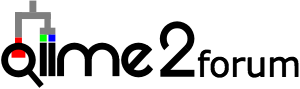# ggplot Relative abundance_Out of 100%

Hello everyone,

I have one question regarding the relative frequency plot. I am trying to perform a stacked plot for my microbiome data, but in y axis (relative frequencies) I get much more than 100 and I don't know why it goes way higher than 100 in the plot. I have read other posts but I couldn't figured it out. I really appreciate if you can help me out, please.

The dataset:
L2_16S_R2.csv (5.5 KB)

The codes are:
library(tidyverse)
library(glue)
library(ggtext)
library(patchwork)
library(reshape2)
library(ggtext)
################# L2_16S #################

View(pc)
#convert data frame from a "wide" format to a "long" format
pcm = melt(pc, id = c("Vineyard"))
View(pcm)
str(pcm)
pcm %>%
group_by(Vineyard, variable) %>%
summarize(value = sum(value), .groups="drop") %>%
group_by(Vineyard, variable) %>%
summarize(mean_value = mean(value), .groups="drop") %>%
mutate(variable=str_replace(variable,
"(.)_unclassified", "Unclassified \1"),
variable = str_replace(variable,
"^(\S
)\$", "\1")) %>%
ggplot(aes(x=Vineyard, y=mean_value, fill= variable)) +
geom_col() +
labs(x = NULL,
y = "Mean relative abundance (%)") +
theme_classic() +
theme(axis.text.x = element_markdown(),
legend.text = element_markdown())

ggsave("L2_16S.tiff", width =5, height = 4)

The graph that I get is:

Thank you

Hi!
I am not very good in R, so can not comment on your code, but what I noticed is that your dataset looks strange for relative abundances as %. For example, summ of all features in a sample is not equal to 100%, but summ of all samples per feature is equal. I think you made an error when converted absolute abundances to relative abundances. Summ of all features in a sample should be equal 100, not the summ of all samples per feature.

1 Like

Hi,

You may want to check your data and see if it is correct. The data doesn't look like count data: all numbers have seven digits and they're smaller than ten. If the data has been subjected to total sum scaling (tss), then the row sums should be 1 or 100% but they are not.

Anyway, assuming numbers in the csv file are sequence counts, we can plot the data by running the following code:

``````# import data

# tidy data
pcm <- pc %>%
# add unique sample ids so that we can normalize data later
mutate(sample_id = 1:nrow(pc), .after = Vineyard) %>%
melt(id = c("Vineyard", "sample_id")) %>%
group_by(sample_id) %>%
# normalize data: convert counts to percentage (total sum scaling, tss)
mutate(value_tss = 100 * value/sum(value)) %>%
ungroup() %>%
# the following 2 lines of code calculate the mean relative abundance of each phylum
group_by(Vineyard, variable) %>%
summarize(value_tss_mean = mean(value_tss)) %>%
# I'm not familar with stringr, so I use gsub for the string manipulation
mutate(
variable = paste0("*", variable, "*"),
variable = gsub("\\*Bacteria_unclassified\\*", "Unclassifed \\*Bacteria\\*", variable)
)

# make plot
ggplot(pcm, aes(x = Vineyard, y = value_tss_mean, fill = variable)) +
geom_col() +
labs(
x = NULL,
y = "Mean relative abundance (%)",
fill = "Phylum"
) +
theme_classic() +
theme(
legend.text = element_markdown()
)
``````

3 Likes

I apologize for my delay respond.
Thanks so very much! You are right. The data were not correct and I fixed it!!
The codes works perfectly.